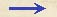Discorsi Propositions 1/04-th-04 Discorsi Proposition1/04-th-04THEOREMA IV, PROPOSITIO IV. THEOREM IV, PROPOSITION IVSi duo mobilia ferantur motu aequabili, inaequali tamen velocitate, spatia temporibus inaequalibus AB ipsis peracta habebunt rationem compositam ex ratione velocitatum et ex ratione temporum. If two particles are carried with uniform motion, but each with a different speed, the distances covered by them during unequal intervals of time bear to each other the compound ratio of the speeds and time intervals. Mota sint duo mobilia E, F motu aequabili, et ratio velocitatis mobilis E ad velocitatem mobilis F sit ut A ad B; temporis vero quo movetur E, ad tempus quo movetur F, ratio sit ut C ad D: dico, spatium peractum ab E cum velocitate A in tempore C, ad spatium peractum ab F cum {20} velocitate B in tempore D, habere rationem compositam ex ratione velocitatis A ad velocitatem B et ex ratione temporis C ad tempus D. Sit spatium ab E cum velocitate A in tempore C peractum G, et ut velocitas A ad velocitatem B, ita fiat G ad I; ut autem tempus C ad tempus D, ita sit I ad L: constat, I esse spatium quo movetur F in tempore eodem in quo E motum est per G, cum spatia G, I sint ut velocitates A, B.Et cum sit ut tempus C ad tempus D, ita I ad L; sit autem I spatium quod conficitur A mobili F in tempore C; erit L spatium quod conficitur ab F in tempore D cum velocitate B. Ratio autem G ad L componitur ex rationibus G ad I et I ad L, nempe ex rationibus {30} velocitatis A ad velocitatem B et temporis C ad tempus D: ergo patet propositum. Let the two particles which are carried with uniform motion be E and F and let the ratio of the speed of the body E be to that of the body F as A is to B; but let the ratio of the time consumed by the motion of E be to the time consumed by the motion of F as C is to D. Then, I say, that the distance covered by E, with speed A in time C, bears to the space traversed by F with speed B in time D a ratio which is the product of the ratio of the speed A to the speed B by the ratio of the time C to the time D. For if G is the distance traversed by E at speed A during the time-interval C, and if G is to I as the speed A is to the speed B; and if also the time-interval C is to the time-interval D as I is to L, then it follows that I is the distance traversed by F in the same time that G is traversed by E since G is to I in the same ratio as the speed A to the speed B.And since I is to L in the same ratio as the time-intervals C and D, if I is the distance traversed by F during the interval C, then L will be the distance traversed by F during the interval D at the speed B. But the ratio of G to L is the product of the ratios G to I and I to L, that is, of the ratios of the speed A to the speed B and of the time-interval C to the time-interval D. Q. E. D.Discorsi Propositions 1/04-th-04 Discorsi Proposition1/04-th-04